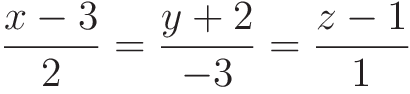Select Page

# MCQ in English Answers for CBSE Maths 12 Science Three Dimensional Geometry

MCQ in English Answers for CBSE Maths 12 Science Three Dimensional Geometry to enable students to get Answers in a narrative video format for the specific question.

Expert Teacher provides MCQ Answers for CBSE Maths 12 Science Three Dimensional Geometry through Video Answers in English language. This video solution will be useful for students to understand how to write an answer in exam in order to score more marks. This teacher uses a narrative style for a question from Three Dimensional Geometry not only to explain the proper method of answering question, but deriving right answer too.

Please find the question below and view the Answer in a narrative video format.

Question:

## Similar Questions from CBSE, 12th Science, Maths, Three Dimensional Geometry

Question 1 : Find the equation of the plane with intercept 3 on the y-axis and parallel to ZOX plane. (View Answer Video)

Question 2 : Find the intercepts cut off by the plane 2x + y - z = 5. (View Answer Video)

Question 3 : Find the vector and cartesian forms of the equation of the plane passing through the point (1, 2, -4) and parallel to the linesandAlso, find the distance of the point (9, -8, -10) from the plane thus obtained. (View Answer Video)

Question 4 : The cartesian equation of a line is, write its vector form. (View Answer Video)

Question 5 : Find the distance of the pointfrom the plane 3x + y - z + 2+0 measured parallel to the lineAlso, find the foot of the perpendicular from the given point upon the given plane. (View Answer Video)

### Differential Equations

Question 1 : Write the degree of the differential equation :(View Answer Video)

Question 2 : Form the differential equation of equation y = a cos 2x + b sin 2x, where a and b are constant. (View Answer Video)

Question 3 : If m and n are the order and degree, respectively of the differential equationthen write value of m+n. (View Answer Video)

Question 4 : If x cos(a + y) = cos y, then prove thatHence show that(View Answer Video)

### Probability

Question 1 : An experiment succeeds thrice as often as it fails. Find the probability that in the next trials, there will be atleast 3 successes.    (View Answer Video)

Question 2 : A bag contains 4 green and 6 white balls. Two balls are drawn one by one without replacement. If the second ball drawn is white, what is the probability that the first ball drawn is also white?   (View Answer Video)

Question 3 : One card is drawn from a pack of 52 cards. Find the probability of getting a black face card: (View Answer Video)

Question 4 :  Three numbers are selected at random (without replacement ) from first six positive integers. Let X denote the largest of the three numbers obtained. Find the probability distribution of X. Also, find the mean and variance of the distribution.  (View Answer Video)

Question 5 :  A man is known to speak the truth 3 out of 5 times. He throws a die and reports that it is 1. Find the probability that it is actually 1.   (View Answer Video)

### Inverse Trigonometric Functions

Question 1 : Write in the simplest form:(View Answer Video)

Question 2 : Write the principal value of(View Answer Video)

Question 3 : Write the principal value of(View Answer Video)

Question 4 : Evaluate :(View Answer Video)

Question 5 :Then x is equal to : (View Answer Video)